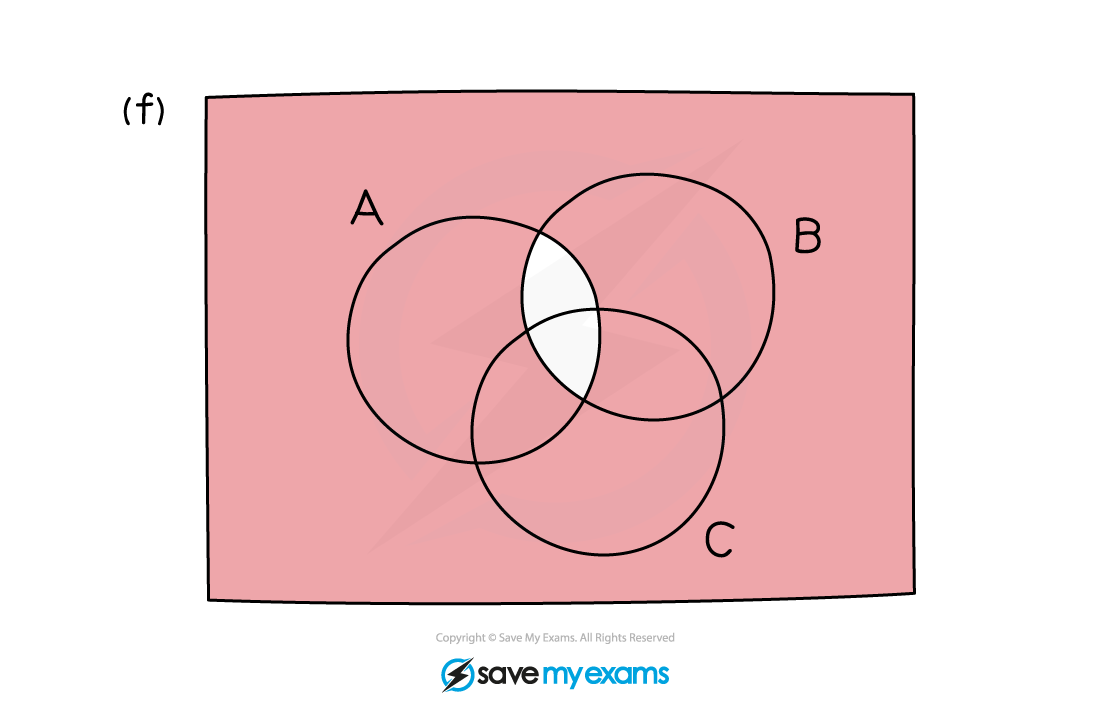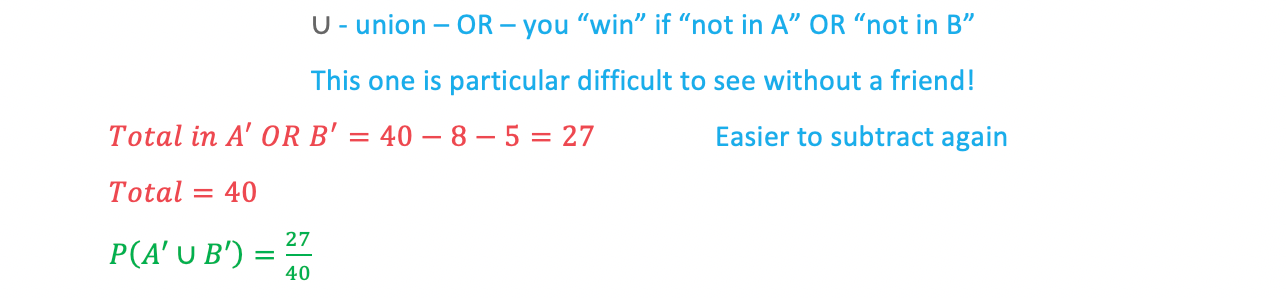# Edexcel IGCSE Maths 复习笔记 6.5.1 Probability - Venn Diagrams

Edexcel IGCSE Maths 复习笔记 6.5.1 Probability - Venn Diagrams

#### What is a venn diagram?

• Venn diagrams allow us to show two (or more) characteristics of a situation where there is overlap between the characteristics
• For example, students in a VI Form can study Biology or Chemistry but there may be students who study both

#### What do I need to know?

• You can be asked to draw a Venn diagram and/or interpret a Venn diagram
• Strictly speaking the rectangle (box) is always essential on a Venn diagram as it represents everything that can happen in the situation
• You may see the letters ε or ζ written inside or just outside the box – this means “the set of all possible outcomes” – basically it just means “everything”!
• The words AND and OR become very important in both drawing and interpreting Venn diagrams
• You will need to be familiar with the symbols ∩ and ∪ – intersection and union, loosely speaking these mean AND and OR (respectively)

1. Drawing a Venn Diagram

• You’ll need a “box” and overlapping “bubbles” depending on how many characteristics you are dealing with
• You will not normally be given all the values for every section in your diagram
• You will be expected to work out missing information in order to complete your Venn diagram
• Remember to consider AND and OR

2. Interpretation

• This is where “not A” (A’) and similar probability that event A does not happen can get confusing
• The symbols ∩ and ∪ are often used here too

#### Exam Tip

Lightly highlighting the part of the Venn diagram you need can help but make sure you can still read the whole diagram for later parts of the question if you do this.

#### Worked Example#### Worked Example

(a)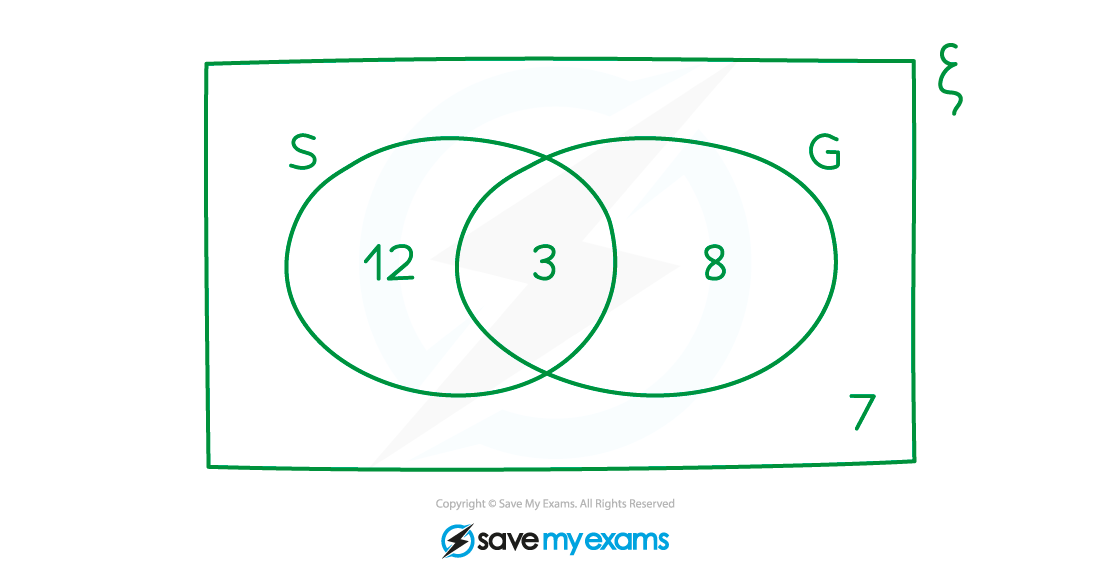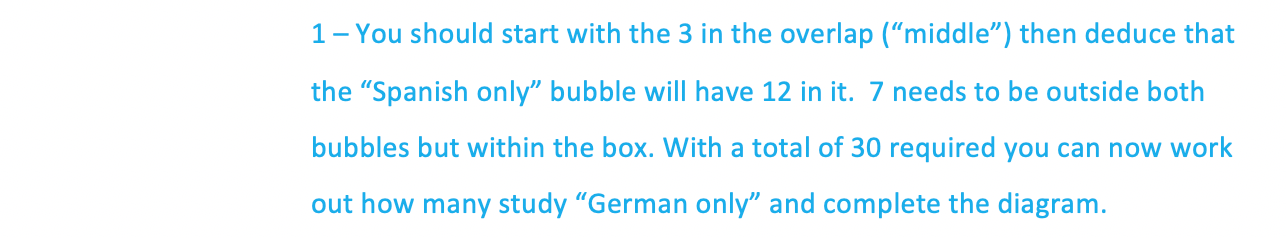#### Worked Example

(b)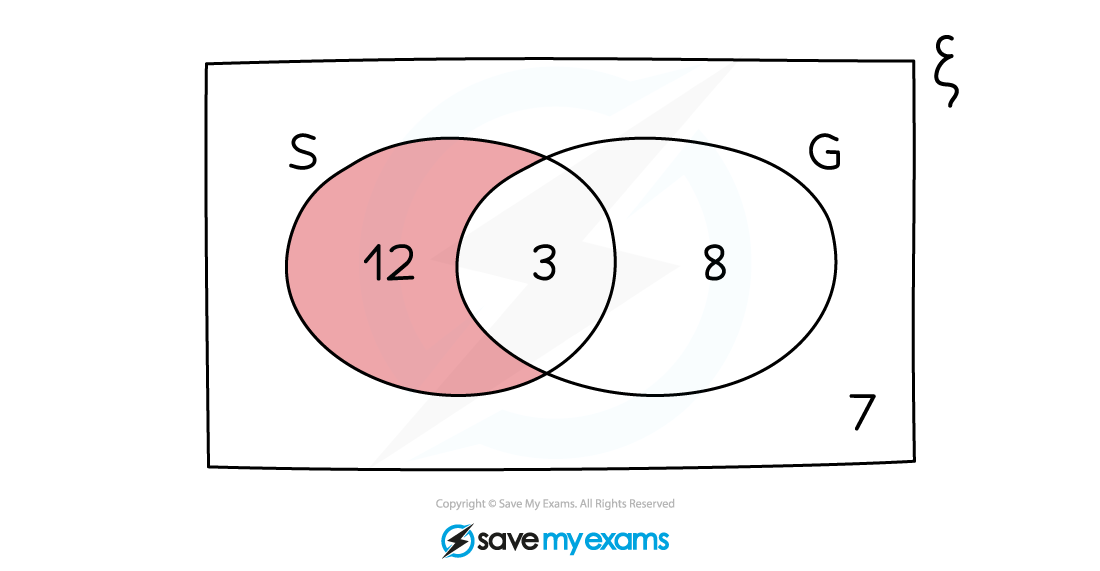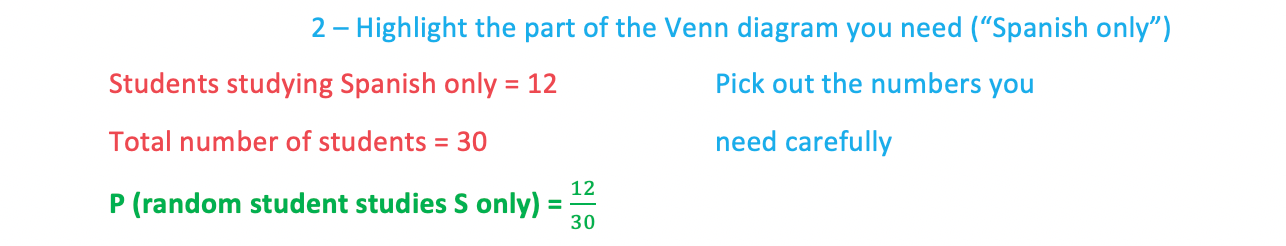#### Worked Example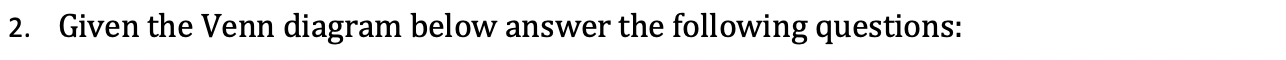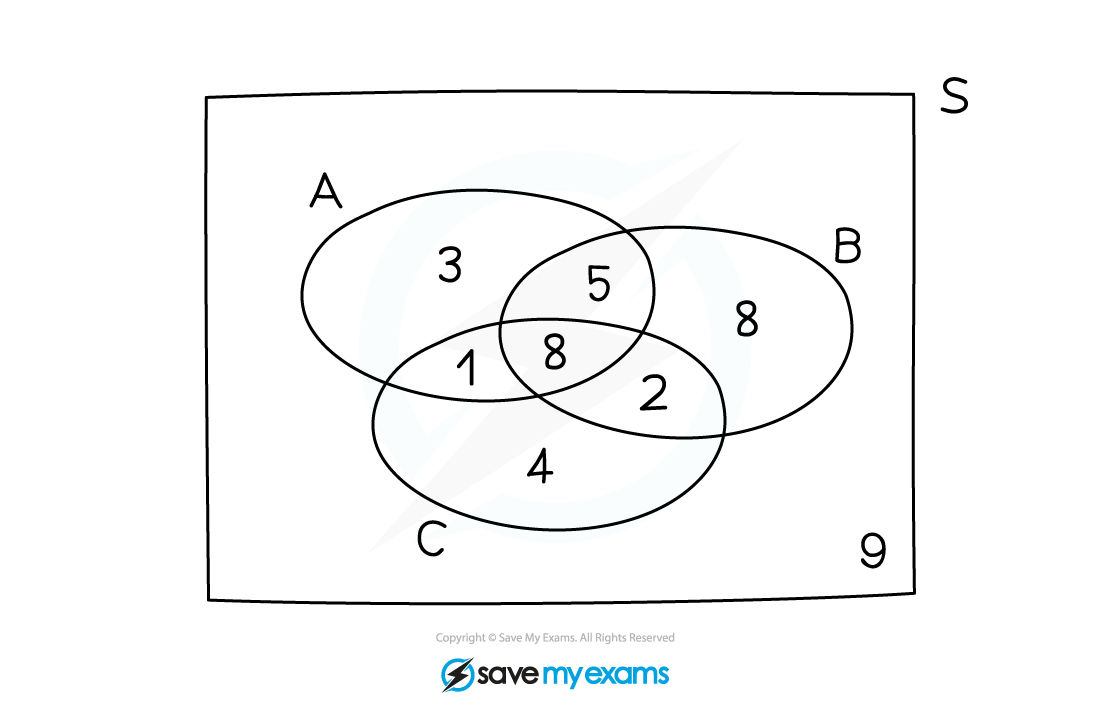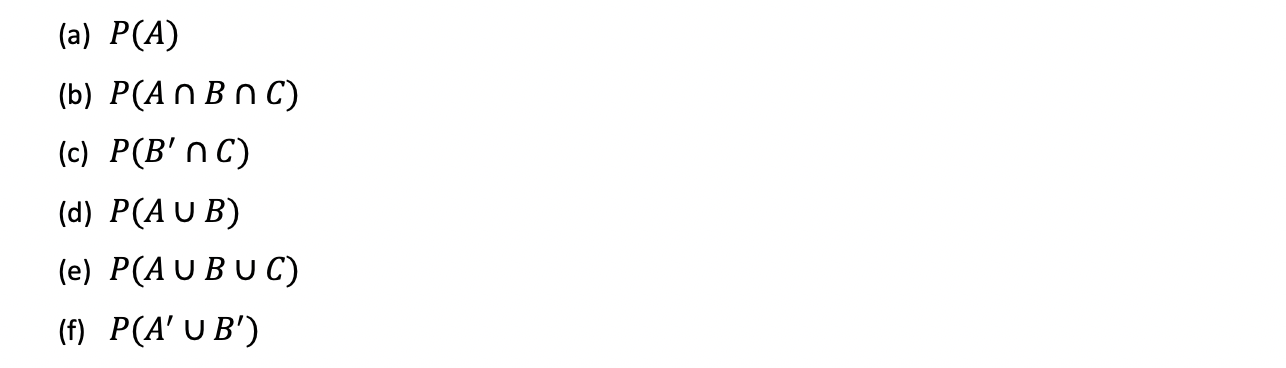#### Worked Example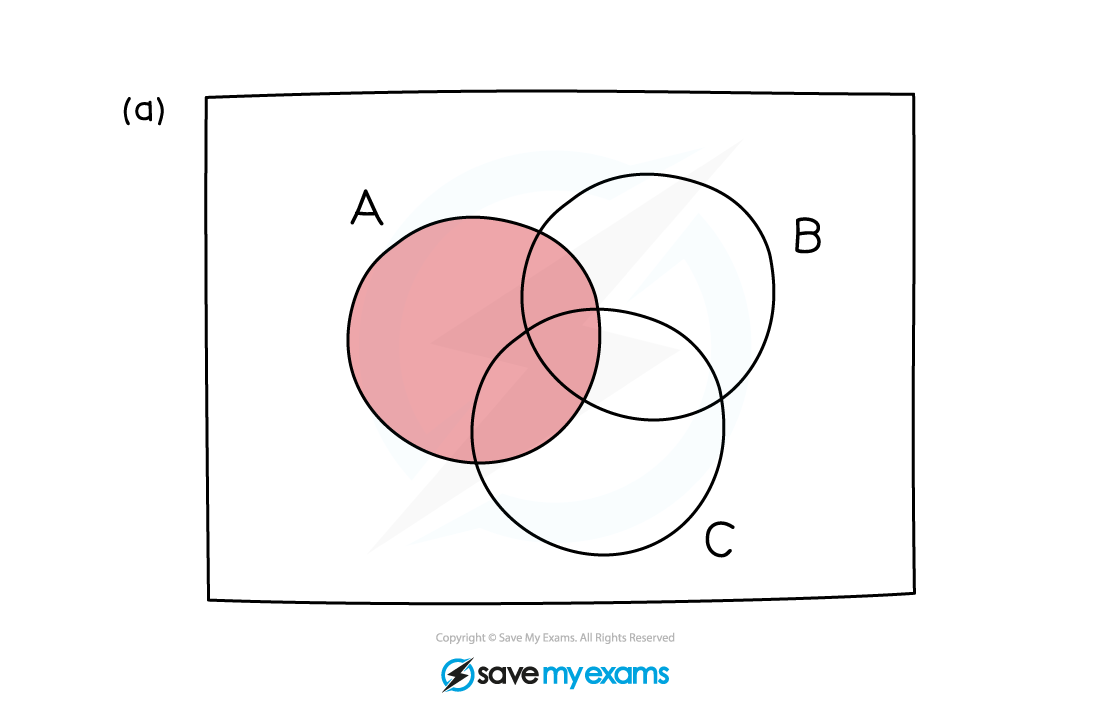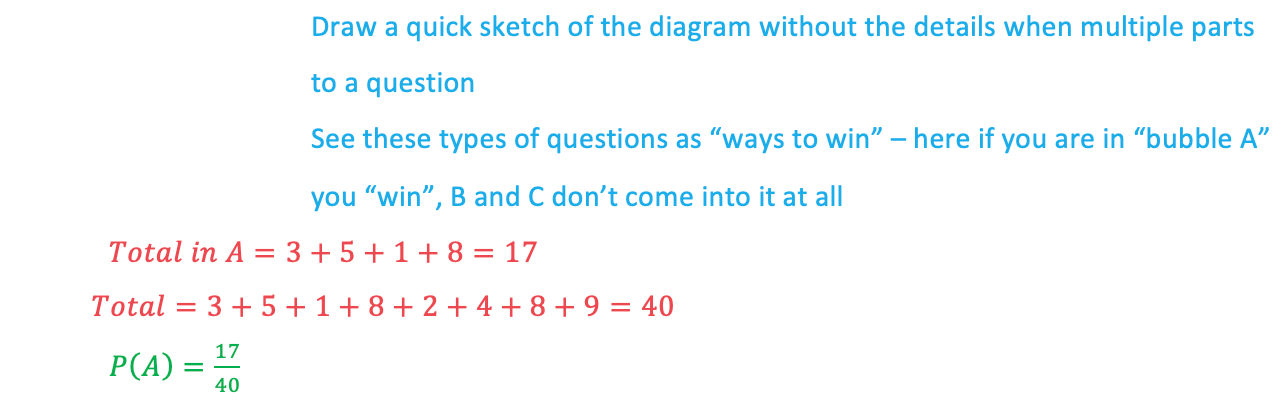#### Worked Example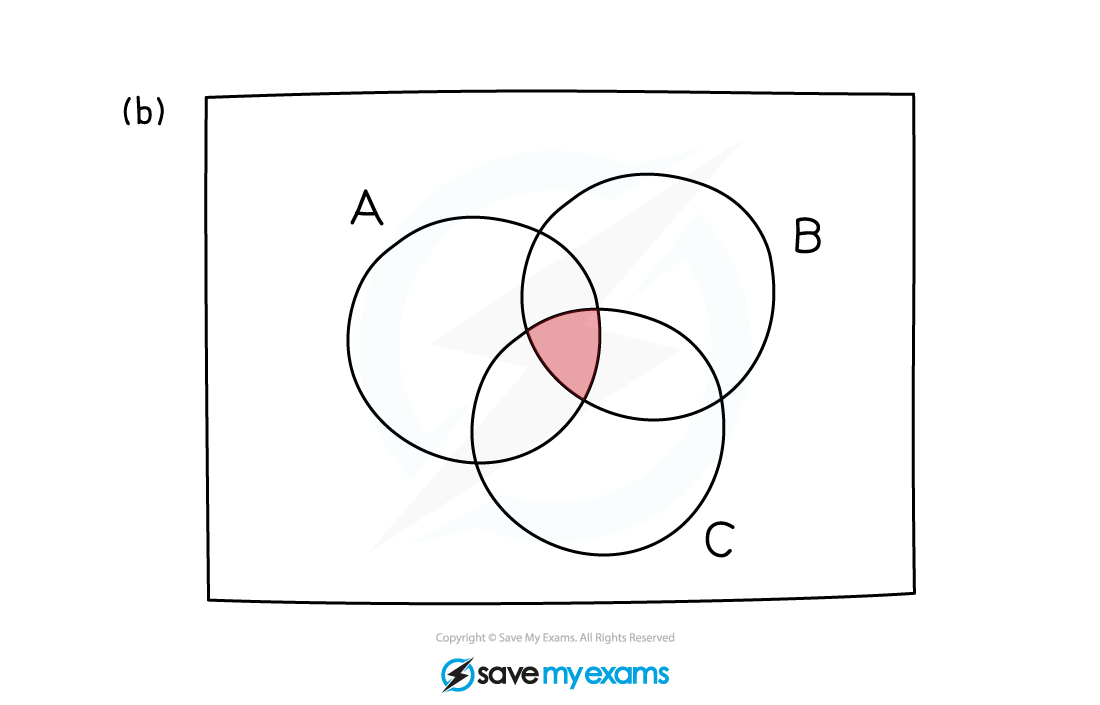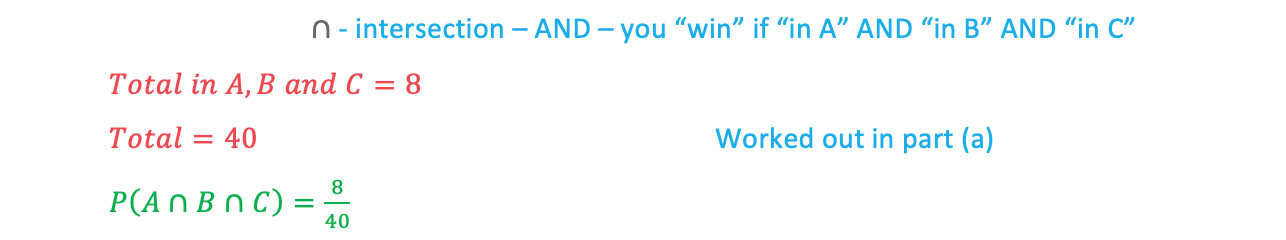#### Worked Example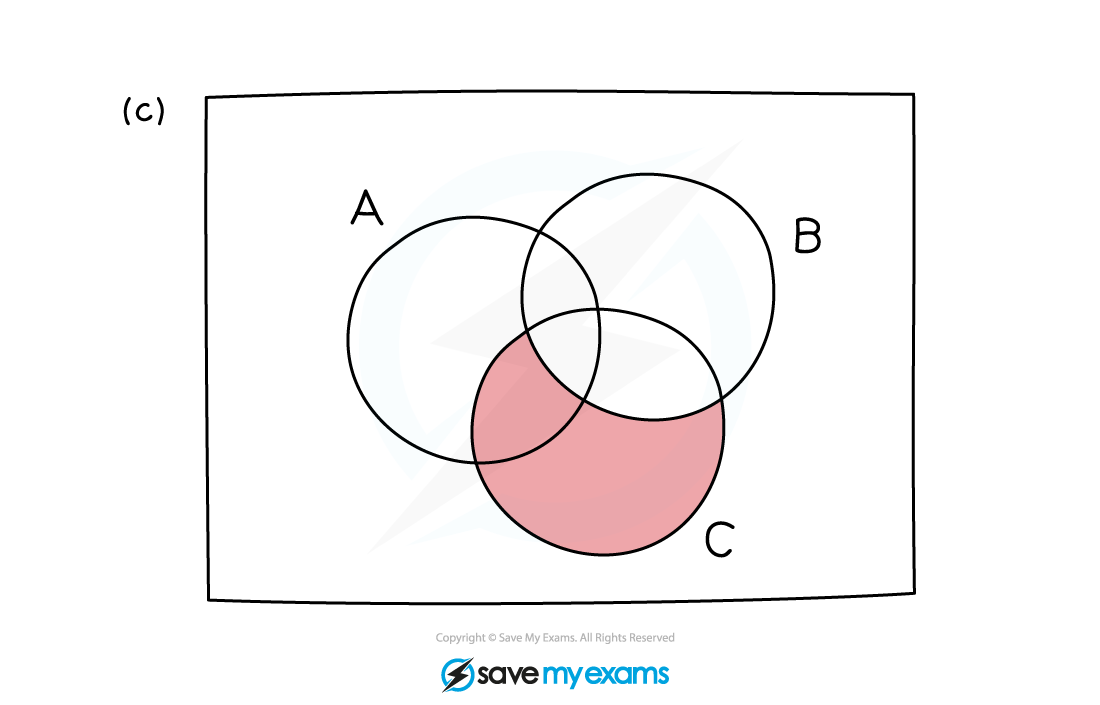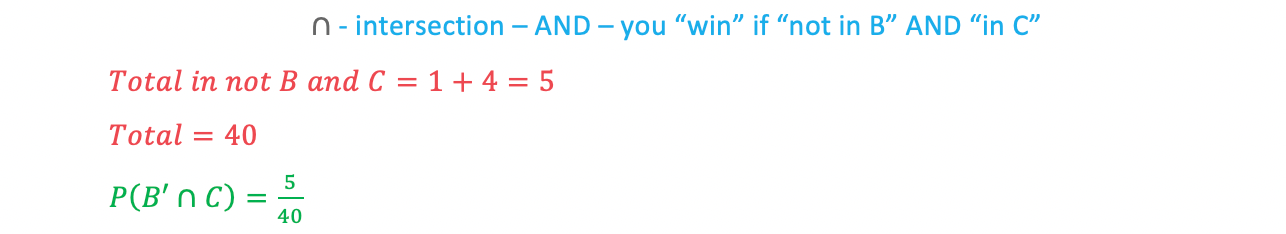#### Worked Example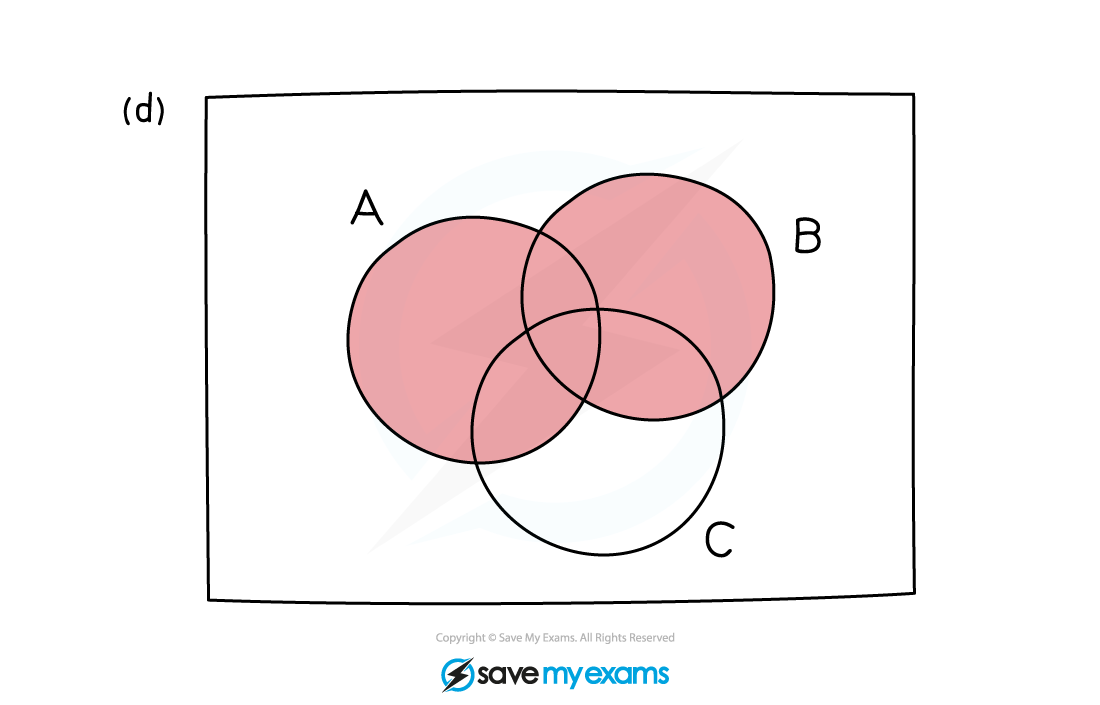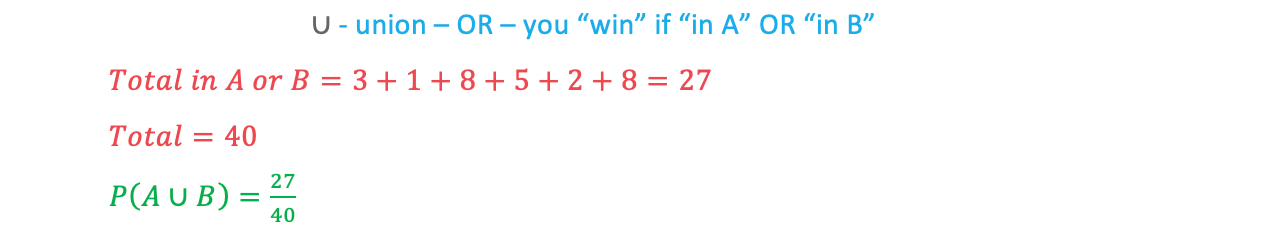#### Worked Example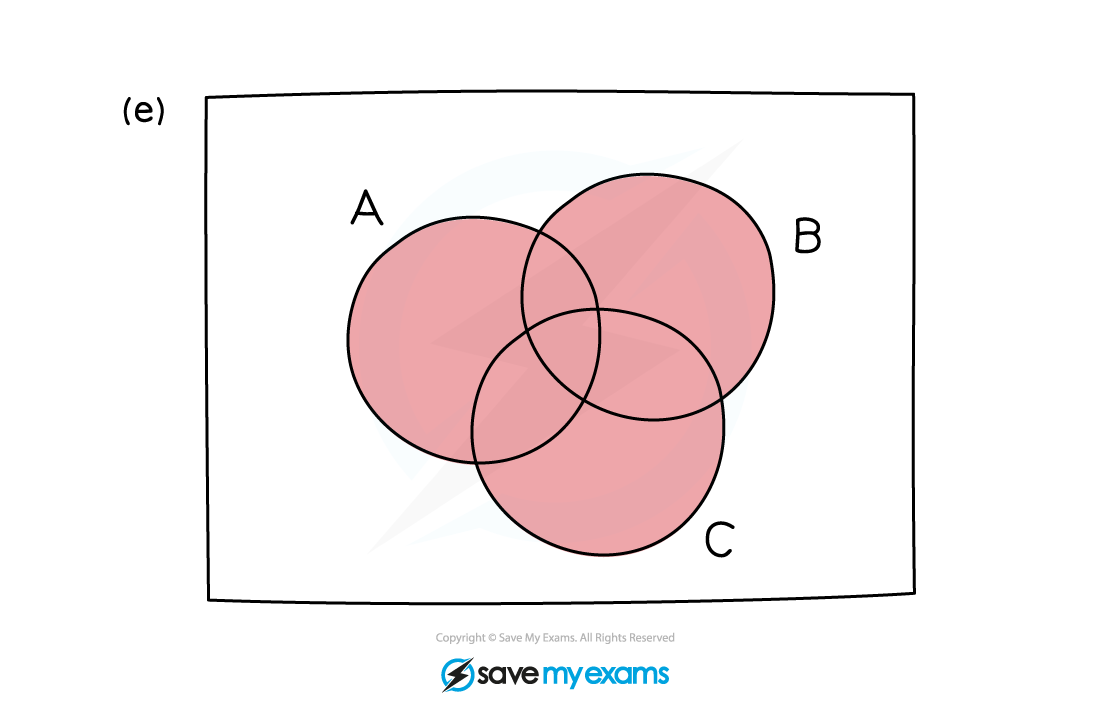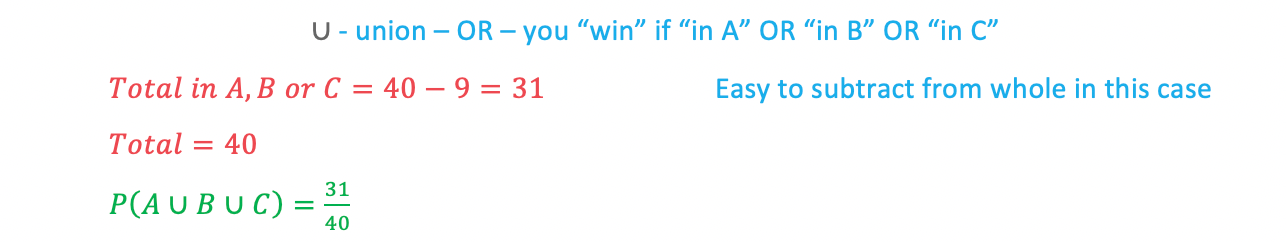#### Worked Example